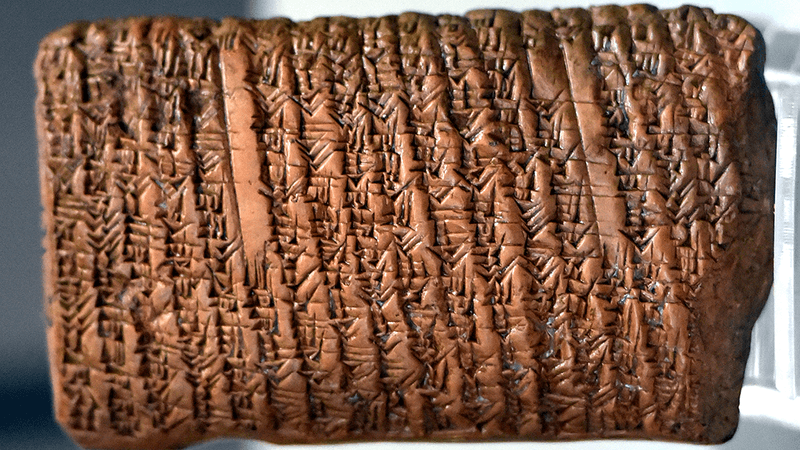HumansPUBLISHED

# Pythagorean Theorem Found On Clay Tablet 1,000 Years Older Than Pythagoras

## It predates Pythagoras by over 1,000 years.64Comments24.6kSharesThe proof is carved into clay.Image credit: Osama Shukir Muhammed Amin FRCP(Glasg) via Wikimedia Commons (CC BY-SA 4.0); rotated, cropped

Study math for long enough and you will likely have cursed Pythagoras's name, or said "praise be to Pythagoras" if you're a bit of a fan of triangles.

But while Pythagoras was an important historical figure in the development of mathematics, he did not figure out the equation most associated with him (a2 + b2 = c2). In fact, there is an ancient Babylonian tablet (by the catchy name of IM 67118) which uses the Pythagorean theorem to solve the length of a diagonal inside a rectangle. The tablet, likely used for teaching, dates from 1770 BCE – centuries before Pythagoras was born in around 570 BCE

Another tablet from around 1800–1600 BCE has a square with labeled triangles inside. Translating the markings from base 60 – the counting system used by ancient Babylonians – showed that these ancient mathematicians were aware of the Pythagorean theorem (not called that, of course) as well as other advanced mathematical concepts.

"The conclusion is inescapable. The Babylonians knew the relation between the length of the diagonal of a square and its side: d=square root of 2," mathematician Bruce Ratner writes in a paper on the topic. "This was probably the first number known to be irrational. However, this in turn means that they were familiar with the Pythagorean Theorem – or, at the very least, with its special case for the diagonal of a square (d= a+ a= 2a2) – more than a thousand years before the great sage for whom it was named."

So why did this get attributed to Pythagoras? No original writing from Pythagoras survives. What we know of him was passed on by others, in particular the Pythagoreans – members of a school he set up in what is now modern-day southern Italy. The school, named the Semicircle of Pythagoras, was secretive, but knowledge learned there or discovered was passed on, and often attributed to the man himself.

"One reason for the rarity of Pythagoras original sources was that Pythagorean knowledge was passed on from one generation to the next by word of mouth, as writing material was scarce," Ratner continued. "Moreover, out of respect for their leader, many of the discoveries made by the Pythagoreans were attributed to Pythagoras himself; this would account for the term ‘Pythagoras’ Theorem’."

Though Pythagoras did not come up with the theory, his school certainly popularized it, and it became associated with him for the next few thousand years, at least.

### ARTICLE POSTED INHumans
•• mathematics,

• maths,

• Babylonians,

• theorem,

• history of science,

• pythagoras